# Lagrangian and Eulerian Description Civil Engineering (CE) Notes | EduRev

## Civil Engineering (CE) : Lagrangian and Eulerian Description Civil Engineering (CE) Notes | EduRev

The document Lagrangian and Eulerian Description Civil Engineering (CE) Notes | EduRev is a part of the Civil Engineering (CE) Course Advanced Solid Mechanics - Notes, Videos, MCQs & PPTs.
All you need of Civil Engineering (CE) at this link: Civil Engineering (CE)

Lagrangian and Eulerian description
In the development of the basic principles of continuum mechanics, a body B is endowed with various physical properties which are represented by scalar or tensor fields, defined either on a reference configuration,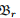or on the current configuration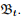In the former case, the independent variables are the position vectors of the particles in the reference configuration, X and time, t. This characterization of the field with X and t as independent variables is called Lagrangian (or material) description. In the latter case, the independent variables are the position vectors of the particles in the current configuration, x and time, t. The characterization of the field with x and t as independent variables is called Eulerian (or spatial) description. Thus, density, displacement and stress are examples of scalar, vector and second order tensor fields respectively and can be represented as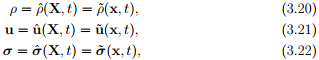where the second equality is obtained by using the relation X = χκ−1 (x, t), which is possible because the function χκ in (3.9) is one to one. Here functions with a hat denote that the independent variables are X and t, while functions with a tilde denote that the independent variables are x and t.

To understand the difference between the Lagrangian description and Eulerian description, consider the flow of water through a pipe from a large tank. Now, if we seed the tank with micro-spheres and determine the velocity of these spheres as a function of time and their initial position in the tank then we get the Lagrangian description for the velocity. On the other hand, if we choose a point in the pipe and determine the velocity of the particles crossing that point as a function of time then we get the Eulerian description of velocity. While in fluid mechanics we use the Eulerian description, in solid mechanics we use Lagrangian description, mostly.

Next, we define what is called as the material time derivative or Lagrangian time derivative or total time derivative and spatial time derivative or Eulerian time derivative. It could be inferred from the above that various variables of interest are functions of time, t and either X or x. Hence, when we differentiate these variables with respect to time, we can hold either X a constant or x a constant. If we hold X a constant while differentiating with time, we call such a derivative total time derivative and denote it by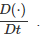. On the other hand if we hold x a constant, we call it spatial time derivative and denote it by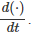. Thus, for the scalar field defined by, say (3.20) we have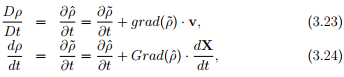where grad(·) stands for the gradient with respect to x, Grad(·) stands for the gradient with respect to X and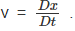. The above equations are obtained by using the chain rule. Similarly, for a vector field defined by (3.21) we have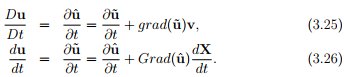Recognize that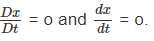Finally, a note on the notation. If the starting letter is capitalized for any of the operators introduced in chapter 2 then it means that the derivative is with respect to the material coordinates, i.e. X otherwise, the derivative is with respect to the spatial coordinates, i.e. x. In the above, we have already used this convention for the gradient operator.

Offer running on EduRev: Apply code STAYHOME200 to get INR 200 off on our premium plan EduRev Infinity!

## Advanced Solid Mechanics - Notes, Videos, MCQs & PPTs

42 videos|61 docs

,

,

,

,

,

,

,

,

,

,

,

,

,

,

,

,

,

,

,

,

,

;